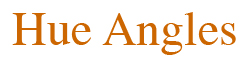## Friday, May 1, 2015

### Equal-Energy White: Does it Illuminate or Obscure?

All illuminants are equal-energy, but some are more equal than others.

Once in a while I have to rant about a theoretical point that requires some mathematical discussion. I apologize in advance to those who expect a minimum of math in this column. But I believe some editorial space is warranted by something that consensus has given an undeserved special status.

We are often cautioned by the CIE not to confuse lights with illuminants: Lights are physically real but illuminants are just lists of numbers juxtaposed with wavelengths. The distinction can at times appear pedantic: Illuminants are derived from physical measurement, aren’t they? Yes, but there is more to say. Some illuminants are more closely tied to physical reality than others. For example, D65 emerged from a principal-component analysis of measured spectra, but nobody has ever actually seen D65 because it is a statistical distillation---like the average family having a fractional number of children. Even farther from physical reality is the equal-energy white illuminant, which is not even a statistical distillation of any real measurements. Yet the CIE has dubbed it “Standard Illuminant E”, it is sometimes called EE, and it has had an interesting history.

For many decades, the equal-energy white illuminant has been a bulwark of color science. Defined simply as a constant-in-wavelength spectral-power distribution, E was used in both the 1931 and 1964 CIE systems to area-normalize the color-matching functions (so E gives the “white” X = Y = Z by definition). It is the adaptation state that some believe we awaken in after a long sleep---and the adaptation state to which all colors are transformed in modern CIE color-appearance models.1 Colorimetrists’ affinity for a unit weighting function has also led to such functions being used to define colorimetric quantities that are not illuminant spectra. A unit weighting function such as heralds EE underlies all discussions of orthogonal color-matching functions from D. L. MacAdam to Matrix-R analysis, and is a hidden part of the structure of principal-component analysis of reflectances. (The principal-component eigenvectors are, after all, orthogonal with respect to the unit weighting function.) I will say no more about these non-illuminant choices here.

All seems well in using the equal-energy white as a preferred spectrum, until one thinks: What if we had adopted the EE illuminant as equal-energy per terahertz of frequency ν  instead of per nanometer of wavelength λ? The colorimetric world as we know it would be quite different numerically. For example, the figure below shows the current equal-energy white illuminant (the horizontal black line) and also the wavelength-density plot of the spectrum that is constant in frequency-density over the visible range (the red curve, normalized to 1 at 550 nm). Relative to the former, the latter emphasizes the short-wavelength end of the spectrum. [The red curve is f(λ) = c/λ2 , derived from ν = c/λ and the equal-energy-in-ν relation f(λ) |dλ| = 1 |dν||.]Of course, looking at wavelength- and frequency-based equal-energy spectra doesn’t exhaust the possibilities. Any positive-definite spectrum g(λ) is a viable equal-energy white in a function of wavelength w(λ) = the integral of g(x) dx from 400nm to λ.  [This is implied by the equal-energy-in-w condition g(λ) |dλ| = 1 |dw|.]  But of all the possible white spectra, wavelength-based EE is the one considered special by color research. To paraphrase from George Orwell’s Animal Farm: All illuminants are equal-energy, but some are more equal than others.

Would some benefit be conferred by re-selecting the domain in which E is equal-energy—perhaps an improved visual performance of principal-component approximations? Regardless of the answer, it may be beneficial to re-frame colorimetry so as not to depend on the primacy of the wavelength domain over, say, frequency. Or perhaps it is enough to be careful that our scientific conclusions don’t depend on E as a special illuminant.